Smartick is a fun way to learn math!May26

# Learn How to Estimate a Sum with Examples

In this post, we will learn to estimate a sum.

Do you know what estimating a sum can help us with? When we have to do an operation mentally, often we do not need the exact result but an estimate of the outcome.

Other times, after we have done an operation on paper and want to verify that the amount we got is reasonable, an estimate of the sum also helps us.

It is quite useful, right? Today we will show you some very simple steps to learn how to estimate a sum.

### How to estimate a sum to the nearest tenth

To estimate an amount to the tenth we have 3 steps to follow:

STEP 1: We round the summands to the nearest tenth. Remember:

• If the number ends in 0, 1, 2, 3 or 4, we round down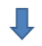• If the number ends in 5, 6, 7, 8 or 9, we round up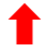STEP 2: We add the rounded numbers

STEP 3: We look at the total amount of rounding. three things can happen:

• If we’ve rounded one amount up and one down, the sum we have obtained is a correct estimation.
• If both summands have been rounded up and the amount of rounding is greater than 5, we subtract 10 from the estimate.
• If both summands have been rounded down and the amount of rounding is greater than 5, we add 10 to the estimate.

Let’s look at an example for each of these three cases:

#### Example 1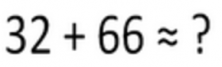• STEP 1: Round the summands.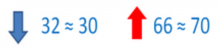• STEP 2: Add the rounded numbers.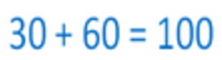• STEP 3: Look at the total amount of rounding. We have rounded one number down and the other up: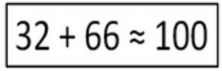The estimate is correct.

#### Example 2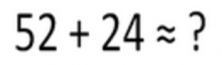STEP 1: Round the summands.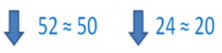STEP 2: Add the rounded numbers.STEP 3: Look at the total amount of rounding.

We have rounded the two summands down. The amount rounded is 2 + 4 = 6.

As 6 is greater than 5, we must add 10 to the estimate: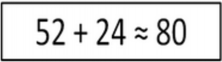#### Example 3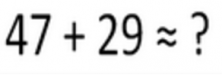STEP 1: Round the summands.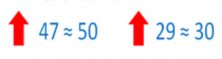STEP 2: Add the rounded numbers.STEP 3: Look at the total amount of rounding.

We have rounded the two summands up. The amount of rounding is 3 + 1 = 4. As 4 is less than 5, the estimate is correct:

###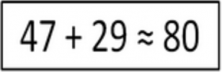How to estimate a sum to the nearest hundred

To estimate a sum to the nearest hundred, we will take the same steps with some changes:

STEP 1: We round the summands to the nearest hundred.

STEP 2: We add the rounded numbers.

STEP 3: We look at the total amount of rounding, three things can happen:

• If we’ve rounded one value up and one down, the sum we have obtained is a correct estimation.
• If both summands have been rounded up and the amount of rounding is greater than 50, we subtract 100 from the estimate.
• If both summands have been rounded down and the amount of rounding is greater than 50, we must add 100 to the estimate.

Let’s look at an example: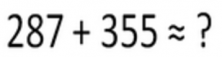STEP 1: We round the summands.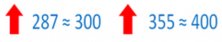STEP 2: We add the rounded numbers.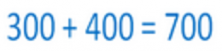STEP 3: We look at the total amount of rounding.

We have rounded both numbers up. The amount of rounding is 13 +45 =58

Because 58 is greater than 50, we should subtract 100 from the result: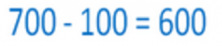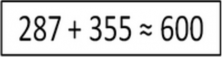It was very easy, right? The next time you need to estimate sums, remember the advice that we have given you today. And remember that you can find exercises to practice this (and much more) on Smartick! If you have not done so, sign up and use the Smartick free trial.

Fun is our brain’s favorite way of learning
Diane Ackerman
Smartick is a fun way to learn math
• 15 fun minutes a day